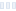•• A
• B
• C
• D
• E
• F
• G
• H
• I
• J
• K
• L
• M
• N
• O
• P
• Q
• R
• S
• T
• U
• V
• W
• X
• Y
• Z

Bandwidth describes the maximum data transfer rate of a network or Internet connection. It measures the amount of data that can be carried from one point to another in a given time period (usually a second). Network bandwidth is usually expressed in bits per second (bps); modern networks typically have speeds measured in the millions of bits per second (megabits per second, or Mbps) or billions of bits per second (gigabits per second, or Gbps). In internet of things era, bandwidth is also expressed in Terra bits per second (Tbps).Stay up-to-date with latest news and special offers!